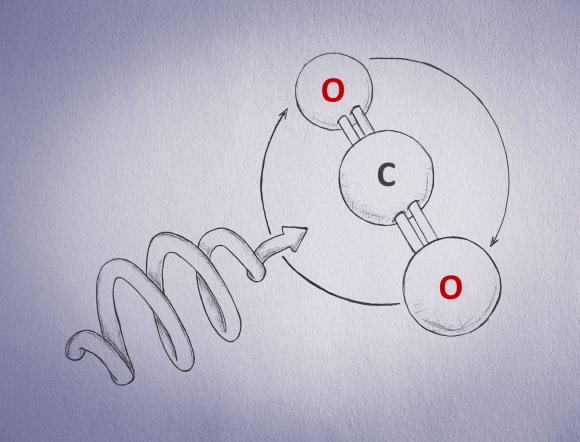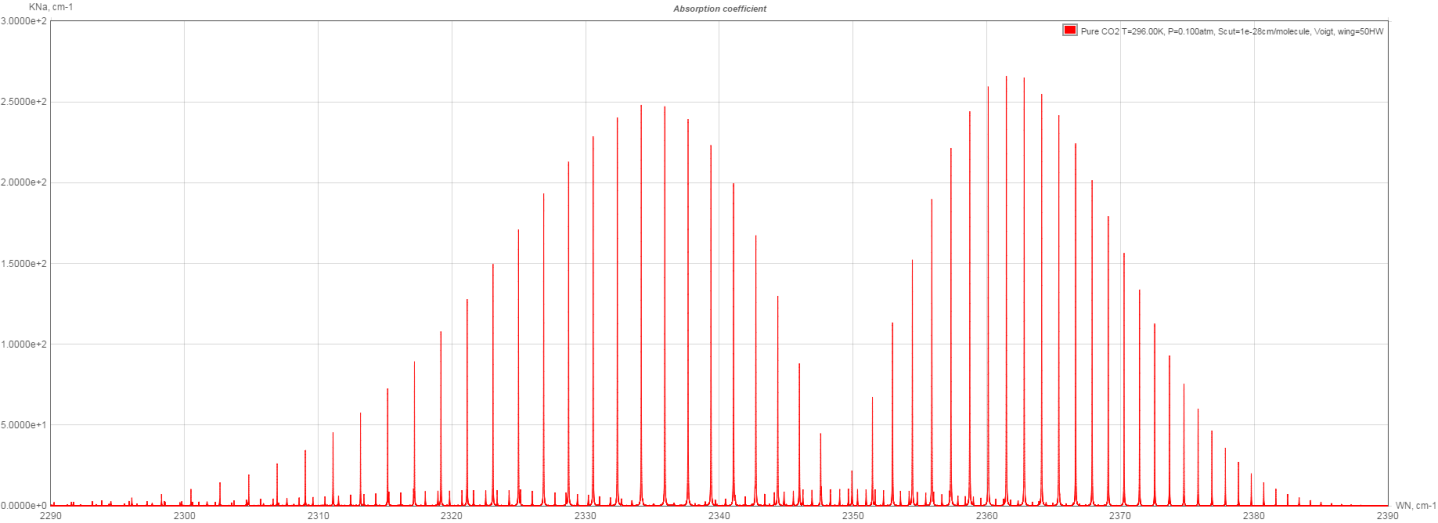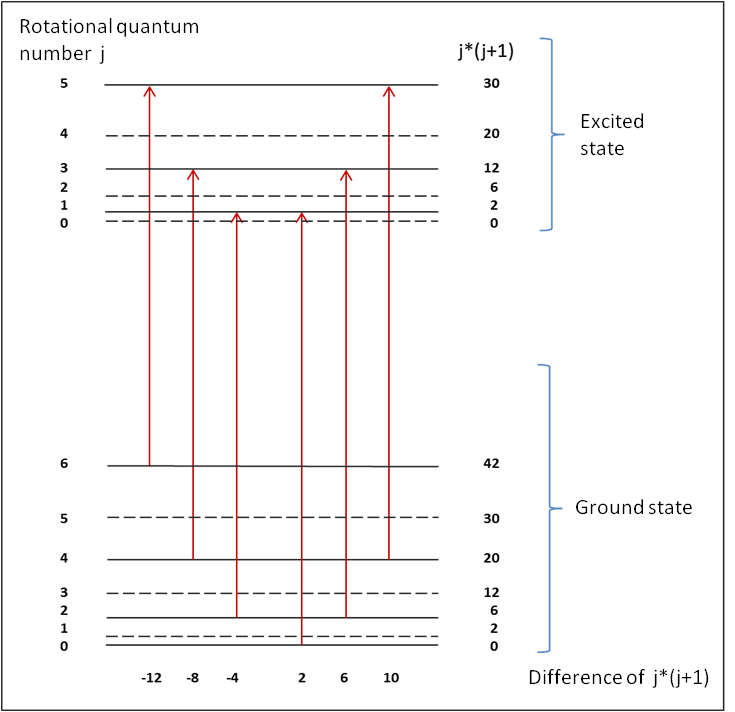5 Rates

# Infrared spectrum of carbon dioxide

## Infrared spectrum of carbon dioxide

Carbon dioxide gives many drinks their refreshing taste. It is also the number one greenhouse gas. In this blog post we take a look at how the infrared spectrum of CO2 is formed and which role quantum physics plays in this.

If we could look at CO2 gas under a microscope – although a microscope with the required specifications will probably never exist – we would see the following:

• The CO2 molecules move around at an average speed which is approximately the speed of sound in the gas: this is about 270 m/s at room temperature.
• The higher the pressure and therefore the number of molecules per volume, the more frequently these molecules collide.
• Most molecules rotate and this rotation is quantized. Therefore, only certain rotational velocities may occur.
• Some molecules vibrate with their deformation vibration. Other normal modes of vibration of the CO2 molecule virtually do not occur because at room temperature there is not enough thermal energy available.

Now we will irradiate CO2 with infrared light and determine the absorption spectrum in the spectral range of the antisymmetric stretching vibration at 2349 cm-1. In contrast to the previous blog post “Quantum physics at work: Infrared spectroscopy”, in which this spectrum was shown at normal pressure, here we will assume a lower gas pressure of 0.1 atm.Fig. 1: A CO2 molecule absorbs a suitable photon whose spin matches the direction of rotation of the molecule. This induces the antisymmetric stretching vibration and accelerates the molecules’ rotation.

Infrared photons with the suitable wave numbers induce the antisymmetric stretching vibration of the CO2 molecules, and either accelerate or slow down their rotation. The photon spin – particularly the direction of rotation of the photon – is responsible for this acceleration or deceleration. Here you can imagine the photon as vibrating in a corkscrew type of movement as shown in Fig. 1, whereby the proportions cannot be adequately shown because the wavelength of the photon is around 10,000 times larger than the CO2 molecule. If the photon’s spin direction matches the direction of rotation of the molecule then the rotational quantum number of the molecule increases by one, otherwise this number decreases by one. The photons absorbed by the molecules are then missing in the infrared light behind the sample, resulting in the absorption spectrum as shown in Fig. 2.Fig. 2: Rotational vibration spectrum for the antisymmetric stretching vibration of CO2 at 0.1 atm pressure and 23 °C. Source: hitran.iao.ru

When comparing this spectrum to the spectrum at normal pressure, we see the following: The individual absorption lines of the spectrum are considerably slimmer. Emerging from the background we see a second spectrum which is shifted by approximately 13 cm-1 to lower wave numbers. The shape of the envelope of the spectral lines also requires discussion as does the question why the line spacing becomes increasingly narrower the higher the wave numbers are. The following questions arise:

(1) Why are the spectral lines broader under normal pressure than at 0.1 atm?

The reason for this is pressure broadening: The more molecules there are moving around per volume, the more frequently they collide and disturb one another. This results in a broadening of the spectral lines with higher gas pressure.

(2) Why do so many spectral lines occur?

At 2349 cm-1 the molecules are only vibrating and not rotating. However, this line does not occur in the spectrum because the photon absorption must lead to a change in the rotational quantum number. The molecules rotate faster and the rotational quantum number is higher the further the line is away from the antisymmetric stretching vibration, i.e. the basic vibration. The further left of the basic vibration the line is, the more energy is provided by the decrease of the rotational quantum number. Due to energy conservation there is correspondingly less photon energy required to induce the antisymmetric stretching vibration. To the right of the basic vibration the rotational quantum number increases. The further right of the basic vibration, the more energy is required to induce the vibration and increase the rotational quantum number.

The higher the temperature, the more thermal energy there is available to cause the molecules to rotate faster, and lines far from the basic vibration are populated. This means that with increasing temperature, further lines occur at the left and right ends of the spectrum in Fig. 2.

(3) Why is the specific shape of the envelope of the spectral lines occurring?

The Boltzmann distribution defines how the total thermal energy is distributed over all the molecules. Here there are additional quantum effects at play: The molecule rotation is not only quantized but is also degenerate: The larger the rotational quantum number and therefore the distance of the line from the basic vibration, the more possibilities there are to populate this rotational level. This leads to an increase in the height of the lines to the right and the left of the basic vibration until there is not enough thermal energy available to populate all the options. Then the height of the lines experiences a maximum and decreases afterwards.

(4) Why does a second spectrum occur and why is it shifted to lower energies?

The deformation vibration of the CO2 molecule at 667 cm-1 is already thermally obtainable at room temperature, meaning that some of the molecules vibrate even when they are not irradiated with infrared light. Therefore, this second spectrum becomes increasingly populated as the temperature increases and the main spectrum becomes correspondingly weaker. Due to the deformation vibration the average inter-atomic distance in the molecule is slightly increased and therefore the binding force is decreased. If the antisymmetric stretching vibration is additionally induced, then this therefore occurs at slightly lower energies, i.e. wave numbers.

(5) Why is the line spacing of the main spectrum twice as wide as the line spacing of the second spectrum?

The rotational constant determines the distance between the rotational lines. It is inversely proportional to the moment of inertia of the molecule. Due to this, larger and/or heavier molecules show rotational lines which are closer than the rotational lines of smaller, lighter molecules.
The non-distinguishability and the spin zero of the two oxygen atoms as well as the Pauli exclusion principle (named after Wolfgang Pauli, Austrian Nobel-prize winner) explain why the line spacing of the main spectrum in Fig. 2 is four rotational constants wide. With the second spectrum the superposition of the deformation vibration and the antisymmetric stretching vibration result in two shifted spectra with a line spacing of 4 rotational constants each, so that the total rotational line spacing is two rotational constants.

(6) Why does the line spacing become narrower as the wave number increases?

As implied in Question (4), vibrating molecules form what is called an anharmonic oscillator whereby their average inter-atomic distances and binding forces change due to the vibration. This affects the moment of inertia and therefore also the rotational constant. The rotational constant of the vibrating molecule is slightly smaller than the rotational constant of the non-vibrating molecule. This results in the required photon energy for inducing the vibration increasing less with increasing wave numbers. There is also another stretching of the inter-atomic distances as the rotational quantum number increases. This causes the line spacing to the left and right of the antisymmetric stretching vibration to become slightly narrower with increasing distance. However, this effect is barely visible to the human eye.

Fig. 3 shows how the first three lines to the left and right of the antisymmetric stretching vibration are formed. The ground state means no vibration; the excited state means the antisymmetric stretching vibration. For the rotational levels of the two states the rotational quantum number j and the number j*(j+1) are given. This last number is proportional to the rotational energy according to the laws of quantum physics.Fig. 3: The formation of the vibration-rotation spectrum around the antisymmetric stretching vibration of CO₂

In the ground state the even-numbered rotational levels are populated; in the excited state the odd-numbered ones. The reasons for this have already been mentioned in Question (5). They are determined by the properties of the quantum physical wave function of each state. With one exception, the difference of j*(j+1) between the head and end of the arrows representing the change from ground state to excited state increases by four from left to right. The line spacing therefore amounts to four rotational constants. Only at the sign change this amounts to 6 rotational constants. If we multiply the above-mentioned difference with the rotational constant and then add the wave number of the antisymmetric stretching vibration we get the wave number of the corresponding line in the spectrum. A more exact calculation takes into account that the rotational constant in the excited state is slightly smaller than the rotational constant in the ground state. For this reason the rotational levels move closer together in the excited state. This results in a line spacing which becomes narrower as the wave number increases, as explained in Question (6).

## Summary

The CO2 molecule shows a rich infrared spectrum of which we have discussed an important part here. The spectrum shows us the properties of the molecule and the effects quantum physics has on it.

If you are interested in measuring the concentration of carbon dioxide in beverages, Anton Paar’s CarboQC and CboxQC are perfectly suited.

Carbon dioxide in the earth’s atmosphere contributes to global warming because it strongly absorbs infrared radiation in a wide range of the spectrum and therefore reduces the heat emission into space. See here for an introduction to the subject of global warming and carbon dioxide.